# Tuesday  September 2

So we can compare objects for symmetries....
how many?
does the multiplication table for the symmetries look the same in some sense?

What about the symmetries of a Frieze Pattern on a Strip....
Video shown on the Alhambra and symmetries of frieze patterns and wallpaper patterns.

Translations and  Reflections

...|p|q|p|q|p|q|p|q|p|q|p|...

This pattern has vertical axes for reflective symmetries between each p and q... and a translation symmetry taking each letter to the next letter of the same type... and also twice as far, and three times as far , and more....

The same is true for the following pattern:

...|d|b|d|b|d|b|d|b|d|b|d|...

Notice the difference -180 degree Rotations and Translations are symmetries for the next pattern.
...|p|p|p|p|p|...
...|d|d|d|d|d|...

The following pattern also has glide reflection symmetry, for example taking p to b and q to d, etc.
It also has a 180 degree rotational symmetry with center midway between the vertical reflection axes and the letter p and d or q and b.

...|p|q|p|q|p|q|p|q|p|q|p|...
...|d|b|d|b|d|b|d|b|d|b|d|...

What are the possible symmetries of a Frieze Pattern on a Strip....

What about the symmetries of a tiling?

Based on the group of symmetries for these patterns,
there are seven possible distinct types of frieze or
Border Patterns: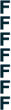translation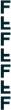horizontal reflection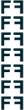vertical reflection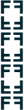reflection + reflection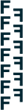glide reflection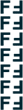rotation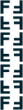reflection + glide reflection

What about the symmetries of a tiling? There are 17 distinct symmetry groups for tiling the plane. They can be described by the following diagrams indicating the symmetries of the figures  as below: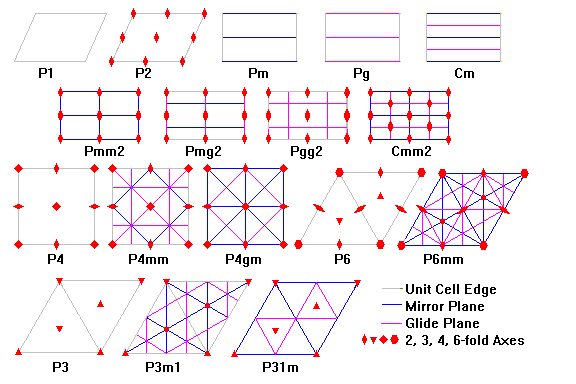or with figures as illustrated by the following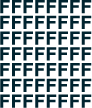translations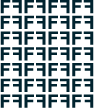reflections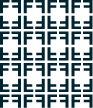reflections + reflections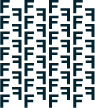glide reflections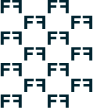reflections + glide reflections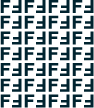rotations (2)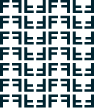reflections + rotations (2)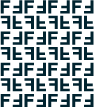rotations (2) + glide reflections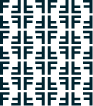rotations (2) + reflections + reflections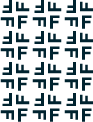rotations (4)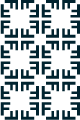reflections + rotations (4)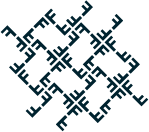rotations (4) + reflections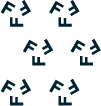rotations (3)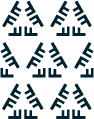reflections + rotations (3)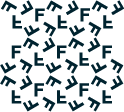rotations (3) + reflectionsrotations (6)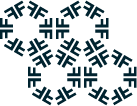reflections + rotations (6)

Do activity on recognizing symmetries in frieze and planar patterns.

One of the features of almost all we have done so far this term, the proofs of the Pythagorean Theorem,  Dissections, Tilings and Symmetry have involved
• Rigid Motions in (or about) the plane.  Also called "Isometries"
• Orientation preserving
• Translations
• Rotations
• Orientation reversing
• Reflections
• Glide reflections

• Classification of Isometries
Next Class...Video : Isometries